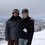# Help: Where has the Kinetic Energy Gone?

I'm looking to fill some apparent gap in knowledge I have. I may have a bit of difficulty in properly conveying my issue, so I hope a discussion can clean it up. Looking at perfectly inelastic collisions, I am somewhat taken back by the fact that kinetic energy is not conserved? Looking at a simple model. Two bodies, one of mass $m$ and the other $M$ are bound for collision with velocities $v$, and 0, respectively. after the collision they join together and continue with velocity $v'$

From conservation of momentum we have: $mv + 0 = (m+M)v'$ , which means that $v' = \frac {m}{( m+M)}v$

At this point lets look at the kinetic energy before and after the collision.

Before Collision: $KE_{before} =\frac {1}{2}mv^2$

After Collision: $KE_{after} = \frac {1}{2}(m+M){v'}^2 = \frac {1}{2}(m+M)(\frac {m}{( m+M)}v)^2 = \frac {1}{2}\frac {m^2}{( m+M)}v^2$

Then taking the ratio of after to before $\frac {KE_{after}}{KE_{before}} = \frac {m}{( m+M)} < 1$ , meaning $KE_{before} > KE_{after}$

So is someone going to tell me the quantity of energy $\frac {1}{2}mv^2 - \frac {1}{2}\frac {m^2}{( m+M)}v^2$ has been lost as heat ( some seemingly divine intervention of the Second Law of Thermodynamics )? I wasn't aware that I was operating under the pretext of entropy in this idealized case? We know absolutely nothing of the internal collision mechaincs that would generate said heat and potential energies of various forms, yet some how this example dictates a quantity of transformed kinetic energy in this collision? For instance, In Newtons Laws ( and even conservation of energy - as far as the models are concerned ) you can simply "turn off" entropy, and the mechanics will yield a limiting case. But here, the limiting case of conserved kinetic energy seems to be impossible to show. So what is going on here?

Any help greatly appreciated.Note by Eric Roberts
1 year, 8 months ago

This discussion board is a place to discuss our Daily Challenges and the math and science related to those challenges. Explanations are more than just a solution — they should explain the steps and thinking strategies that you used to obtain the solution. Comments should further the discussion of math and science.

When posting on Brilliant:

• Use the emojis to react to an explanation, whether you're congratulating a job well done , or just really confused .
• Ask specific questions about the challenge or the steps in somebody's explanation. Well-posed questions can add a lot to the discussion, but posting "I don't understand!" doesn't help anyone.
• Try to contribute something new to the discussion, whether it is an extension, generalization or other idea related to the challenge.

MarkdownAppears as
*italics* or _italics_ italics
**bold** or __bold__ bold
- bulleted- list
• bulleted
• list
1. numbered2. list
1. numbered
2. list
Note: you must add a full line of space before and after lists for them to show up correctly
paragraph 1paragraph 2

paragraph 1

paragraph 2

[example link](https://brilliant.org)example link
> This is a quote
This is a quote
    # I indented these lines
# 4 spaces, and now they show
# up as a code block.

print "hello world"
# I indented these lines
# 4 spaces, and now they show
# up as a code block.

print "hello world"
MathAppears as
Remember to wrap math in $$ ... $$ or $ ... $ to ensure proper formatting.
2 \times 3 $2 \times 3$
2^{34} $2^{34}$
a_{i-1} $a_{i-1}$
\frac{2}{3} $\frac{2}{3}$
\sqrt{2} $\sqrt{2}$
\sum_{i=1}^3 $\sum_{i=1}^3$
\sin \theta $\sin \theta$
\boxed{123} $\boxed{123}$

Sort by:

I think your interpretation is correct. The "lost" kinetic energy is transferred to heat, sound, vibrational modes, etc. It does seem perplexingly convenient, though. Similarly, if you drop a ball from a certain height, you can be confident that ultimately, the change in gravitational potential energy has resulted in an equivalent amount of heat energy added to the local environment. It would be interesting to try to devise a calorimeter machine to confirm these notions empirically.

- 1 year, 8 months ago

Thank you for your response Steven. I see where I was getting mixed up with this concept. I hadn't realized that I was actively putting constraints on the kinetic energy by joining the masses. The simplest of concepts elude my grasp sometimes... I think what was going through my head was something like: calculating a block sliding down a ramp ignoring friction, only to be told by the math at the end that a certain amount of friction was present. I've obviously never encountered that, so it threw me off.

"Similarly, if you drop a ball from a certain height, you can be confident that ultimately, the change in gravitational potential energy has resulted in an equivalent amount of heat energy added to the local environment."

I hadn't actually ever given that concept much thought, thanks for pointing it out!

- 1 year, 8 months ago